#### 期刊菜单

The Applications of Cluster Analysis and Factor Analysis in Performance Evaluations
DOI: 10.12677/SA.2022.111016, PDF, HTML, XML, 下载: 109  浏览: 204  国家自然科学基金支持

Abstract: Programmed in the R software, we use the methods of cluster analysis and factor analysis for performance evaluations. First, we adopt the hierarchical clustering method to get the four different distance hierarchical graphs and the classification table of the performance evaluations. Second, we use factor analysis to choose the teaching factor and the researching factor, use the regression method to calculate the first two factor scores of the teachers, and their two kinds of composite scores. The composite score computed by the first kind of algorithm treats the two factors equally, and the composite score computed by the second kind of algorithm weights the two factors by their variance contribution rates. Finally, we do a comprehensive analysis of teachers’ performance evaluations and obtain that using the hierarchical clustering method when there are no specific quantitative restrictions, and using factor analysis method when there are specific quota restrictions. According to the consistency with the classification by the hierarchical clustering method, we obtain that the factor analysis of the second kind algorithm is better than that of the first kind of algorithm, and use the calculation result of the second algorithm to classify teachers with quota limitation.

1. 引言

2. 聚类分析和因子分析

2.1. 聚类分析

2.2. 因子分析

$X=\mu +AF+\epsilon ,$

$\left(E\left(F\right),E\left(\epsilon \right),Var\left(F\right),Var\left(\epsilon \right),Cov\left(F,\epsilon \right)\right)=\left(0,0,{I}_{m},D,0\right),$

$\underset{i=1}{\overset{p}{\sum }}{\sigma }_{ii}=\underset{i=1}{\overset{p}{\sum }}Var\left({X}_{i}\right)=\underset{j=1}{\overset{m}{\sum }}{g}_{j}^{2}+\underset{i=1}{\overset{p}{\sum }}{\sigma }_{i}^{2}.$

${g}_{j}^{2}$ 反映了公共因子 ${F}_{j}$${X}_{1},{X}_{2},\cdots ,{X}_{p}$ 的影响，可视为公共因子 ${F}_{j}$${X}_{1},{X}_{2},\cdots ,{X}_{p}$ 的总方差贡献。

${\alpha }_{j}=\frac{{g}_{j}^{2}}{\underset{i=1}{\overset{p}{\sum }}Var\left({X}_{i}\right)}=\frac{{g}_{j}^{2}}{p}$Table 1. Expression and characteristics of comprehensive score of factor analysis

3. 实例应用——绩效考评

3.1. 数据收集Table 2. Performance evaluation score table of 25 teachers in a school

3.2. 分析过程

3.2.1. 星图分析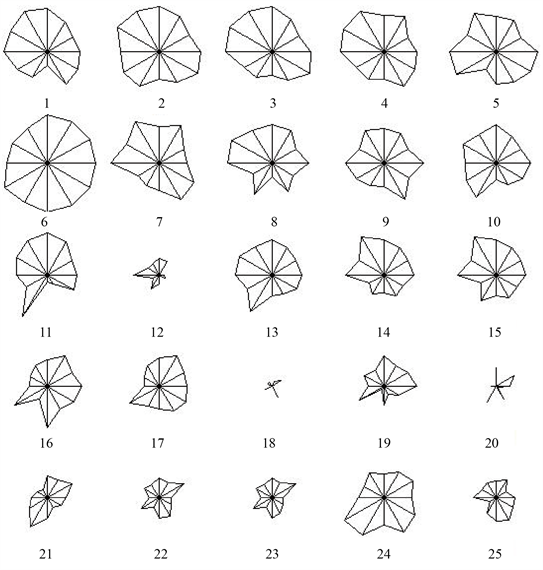Figure 1. Star chart of performance scores of teachers

3.2.2. 聚类分析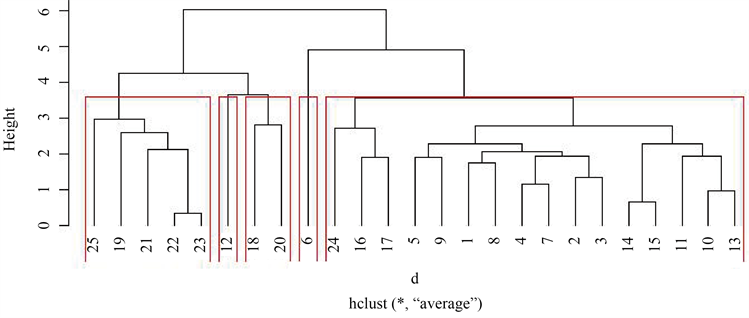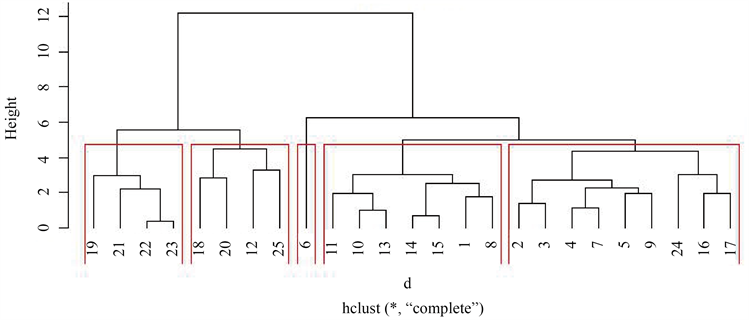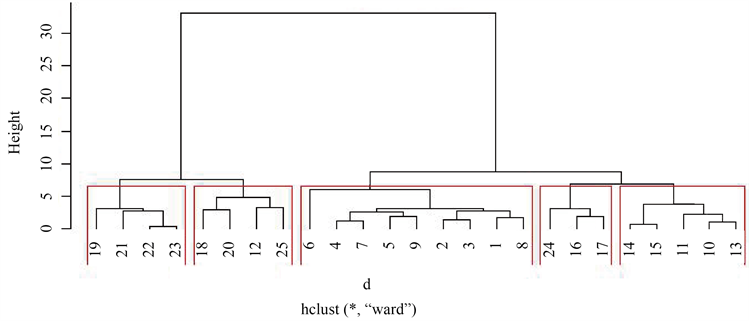Figure 2. Dendrograms of four different distancesTable 3. Classification table of performance evaluation

3.2.3. 因子分析Table 4. Factor loading estimation after rotationTable 5. The comprehensive score of teachers calculated by the first type of comprehensive score algorithm and the comprehensive ranking by F cTable 6. The comprehensive score of teachers calculated by the second type of comprehensive score algorithm and the comprehensive ranking by F c

3.2.4. 综合分析

1) 无具体名额限制时，采用聚类分析法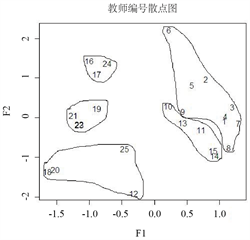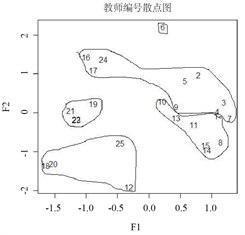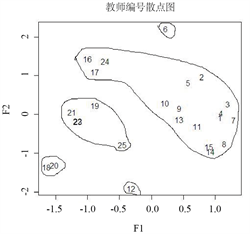类平均法 Mcquitty相似法最长距离法 离差平方和法

Figure 3. Presentation of cluster analysis results on factor score scatter diagram

2) 有具体名额限制时，采用因子分析法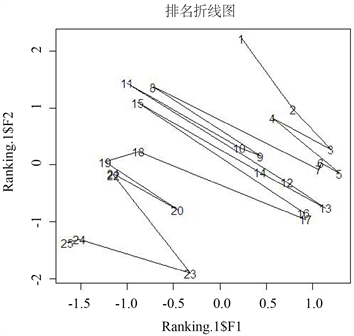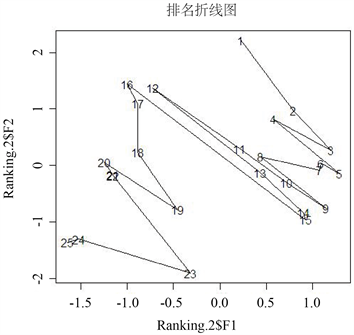第一类算法 第二类算法

Figure 4. Two kinds of algorithm ranking line graph of factor scores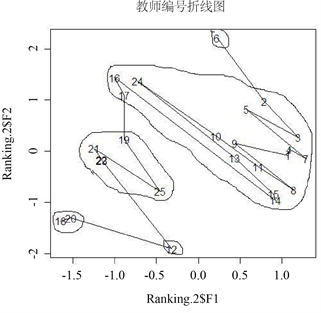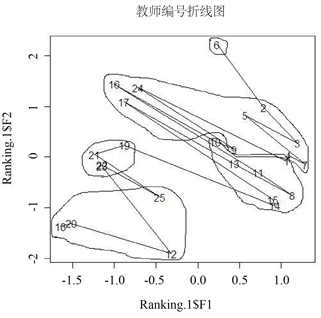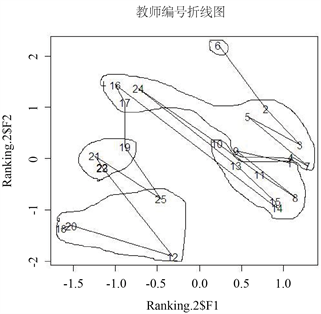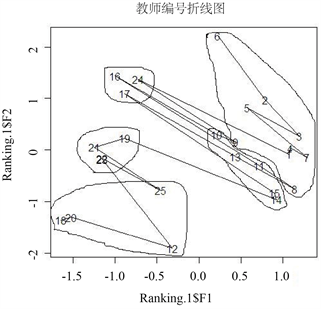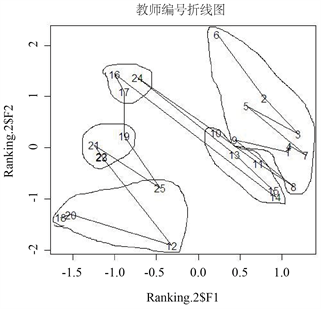Figure 5. Teacher number line chart. The three plots in column 1 (2) are ranked from large to small by ${F}_{1}{}^{c}$ ( ${F}_{2}{}^{c}$ ). The two plots in line 1 (2, 3) are clustered by class average method, Mcquitty similarity method (longest distance method, sum of squares of deviation method)

4. 总结

NOTES

*第一作者。

#通讯作者。

  Johnson, R.A., Wichern, D.W. 实用多元统计分析[M]. 陆璇, 译. 第4版. 北京: 清华大学出版社, 2001.  薛毅, 陈丽萍. 统计建模与R软件[M]. 北京: 清华大学出版社, 2007.  王学民. 多元应用分析[M]. 上海: 上海财经大学出版社, 2009.  杨虎. 多元数据统计分析[M]. 北京: 高等教育出版社, 2013.  王萍萍. 中国农村贫困的地区聚类分析——兼论分地区反贫政策选择[J]. 调研世界, 1999(12): 5-8.  吕岩威, 李平. 一种加权主成分距离的聚类分析方法[J]. 统计研究, 2016, 33(11): 102-108.  沈小云, 衣俊艳. 面向聚类分析的自适应弹性网络算法研究[J]. 计算机工程与应用, 2017, 53(9): 175-183.  李璞, 屈鑫乙, 王迪. 高校教师工作生活质量测量研究[J]. 高教发展与评估, 2018, 34(3): 48-56+66+115.  李欢,熊梦莹, 聂斌, 杜建强, 周丽, 黄强. 融合因子分析的随机森林研究[J]. 计算机工程与应用, 2019, 55(23): 125-130.  钱耀军. 海南与其他经济特区间经济社会发展水平差异测度研究——基于动态因子分析[J]. 调研世界, 2019(4): 61-65.  王诺斯, 彭绪梅, 徐晗. 高校兼职教师教学能力提升路径研究[J]. 高教发展与评估, 2019, 35(4): 53-65+80+112.  林海明, 林敏子. 主成分分析法与因子分析法应用辨析——兼与《我国上市公司赢利能力与资本结构的实证分析》一文作者商榷[J]. 数量经济技术经济研究, 2004(9): 155-160.  林海明, 张文霖. 主成分分析与因子分析的异同和SPSS软件——兼与刘玉玫、卢纹岱等同志商榷[J]. 统计研究, 2005(3): 65-69.  林海明. 因子分析应用中一些常见问题的解析[J]. 统计与决策, 2012(15): 65-69.  王学民. 对主成分分析中综合得分方法的质疑[J]. 统计与决策, 2007(8): 31-32.  苏为华. 多指标综合评价理论与方法问题研究[D]: [博士学位论文]. 厦门: 厦门大学, 2000.  冯根福, 吴林江. 我国上市公司并购绩效的实证研究[J]. 经济研究, 2001(1): 54-61+68.  熊艺, 马朝东. 基于R软件的聚类分析方法在教师绩效考核中的应用[J]. 科技资讯, 2007(19): 191-192.